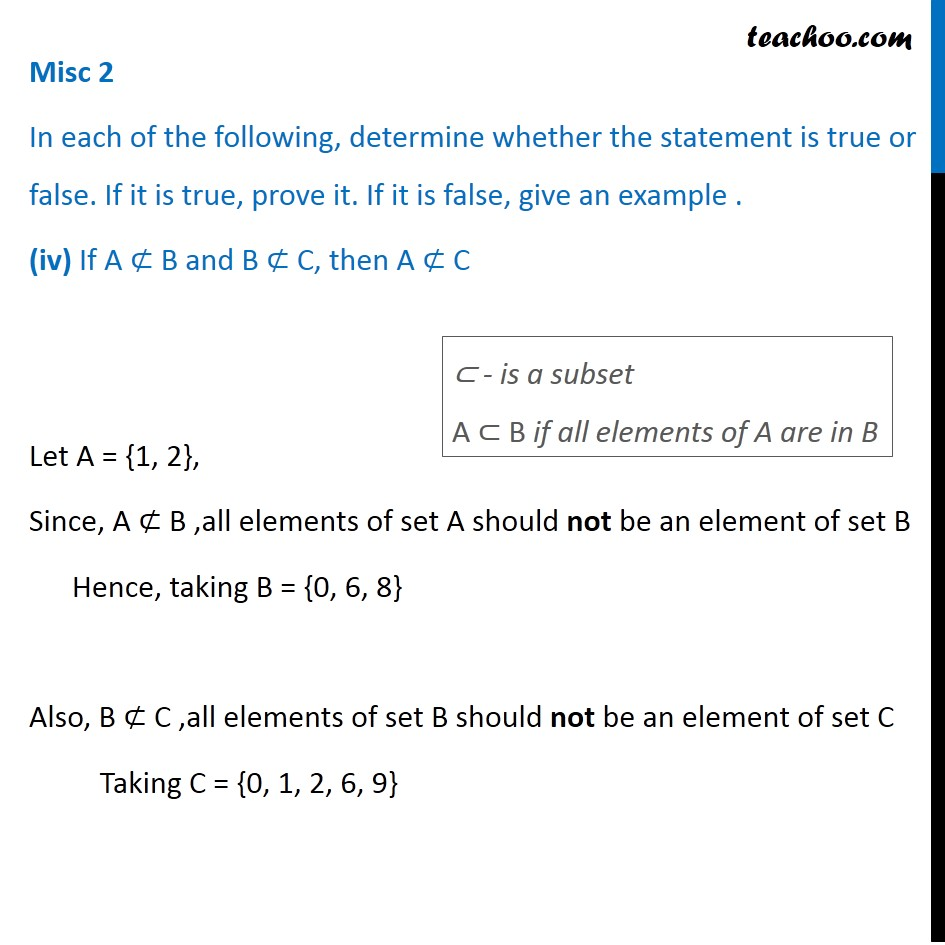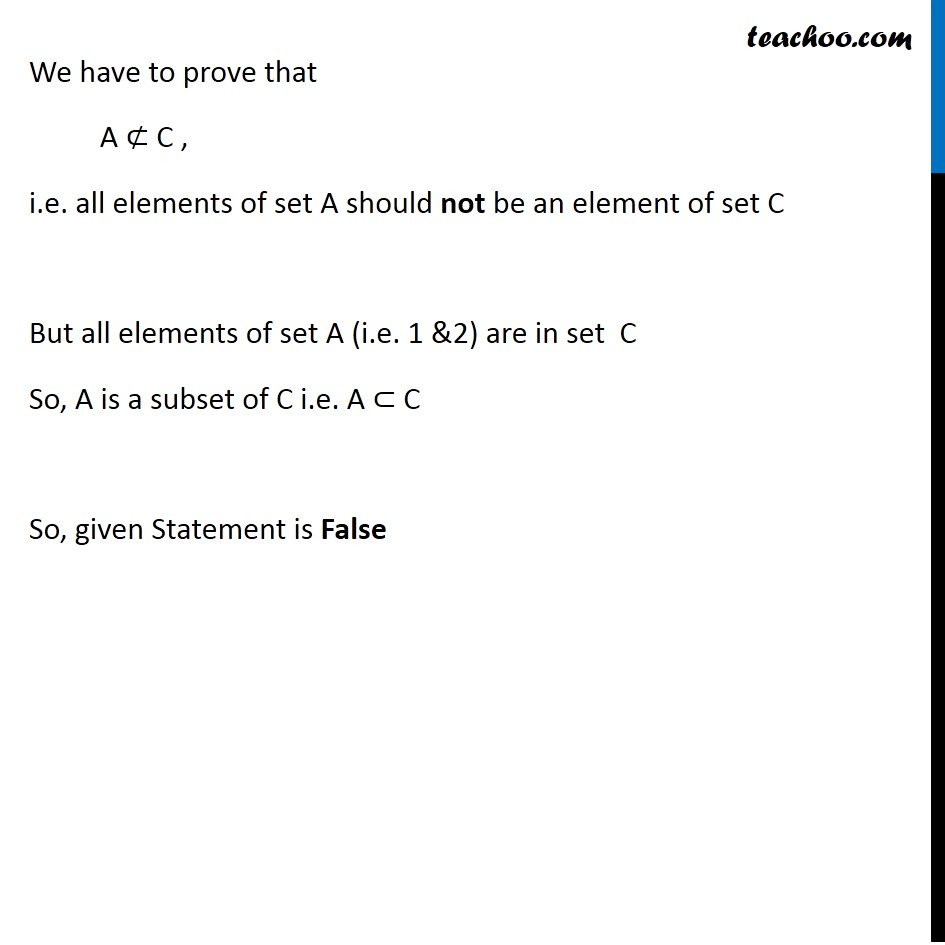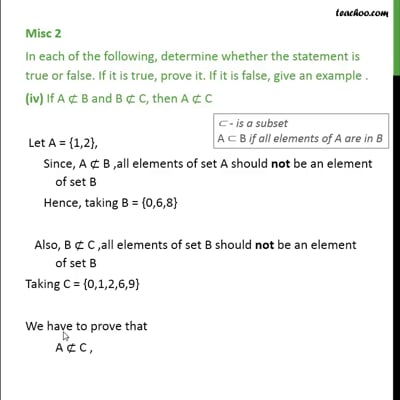Miscellaneous

Chapter 1 Class 11 Sets
Serial order wiseThis video is only available for Teachoo black users

Learn in your speed, with individual attention - Teachoo Maths 1-on-1 Class

### Transcript

Misc 2 In each of the following, determine whether the statement is true or false. If it is true, prove it. If it is false, give an example . (iv) If A ⊄ B and B ⊄ C, then A ⊄ C Let A = {1, 2}, Since, A ⊄ B ,all elements of set A should not be an element of set B Hence, taking B = {0, 6, 8} Also, B ⊄ C ,all elements of set B should not be an element of set C Taking C = {0, 1, 2, 6, 9} ⊂ - is a subset A ⊂ B if all elements of A are in B We have to prove that A ⊄ C , i.e. all elements of set A should not be an element of set C But all elements of set A (i.e. 1 &2) are in set C So, A is a subset of C i.e. A ⊂ C So, given Statement is False